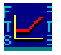Restart to return homeOPTION CALCULATOR:  SENSITIVITIES

The seminal option pricing paper by Black and Scholes 1973 identified the major variables determining the arbitrage free price of an European option on a non dividend paying asset.  This work was extended by Merton to options on a dividend paying underlying asset.  Finally, numerical solutions were discovered for the American option price.

The major pricing variables in these models are:

1. underlying asset price,

2. volatility,

3. maturity,

4. risk free rate,

5. strike price, and

6. dividend yield.

Option sensitivities is made possible from option pricing theory.  This is because the option pricing model (OPM) permits the answer to the question that asks how the option price varies with changes in one of the pricing variables.

In the module to the left you can see the default graph shows how the option price (Y-axis) changes as a function of the underlying asset price (X-axis).  This module lets you select what you plot in the Y- and X- axis, respectively, for the option contract you are currently examining.

On the X-axis are the six drivers of the arbitrage free option price.  This lets you allow these variables to change and observe how one of the dependent variables (e.g. option price) responds to changes in the underlying driver.

On the Y-axis you can plot not only the predicted option price but also other important variables that are used for managing risk and trading options.

These are the hedge parameters:  delta, gamma, vega, theta and rho.

For example, suppose you are currently working with the IBM at-the-money call option; by plotting Option Value against Asset Price, Volatility, and Maturity, you can observe that in each case the predicted option price increases as each of these underlying drivers increase.

To change the plot you can change either the X- or Y-axis variables (or both) and then click on the button labelled Plot.

Reading the Numbers that Correspond to the Graph

A useful additional feature of this module is that by clicking on any point on the graph you can read off what the numerical X- and Y- value is.

Generic Lessons on Hedge Parameters

The following set of lessons let you gain important insights into various aspects of the options sensitivity problems.

What is Delta?

What is Gamma?

What is Vega?

What is Theta?

What is Rho?

OS Financial Trading System, PO Box 11356, Pittsburgh, PA 15238 USA, Phone 1-800-967-9897, Fax 1-412-967-5958, Email fts@ftsweb.com,